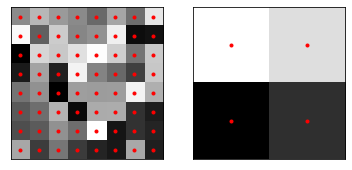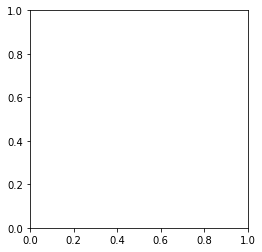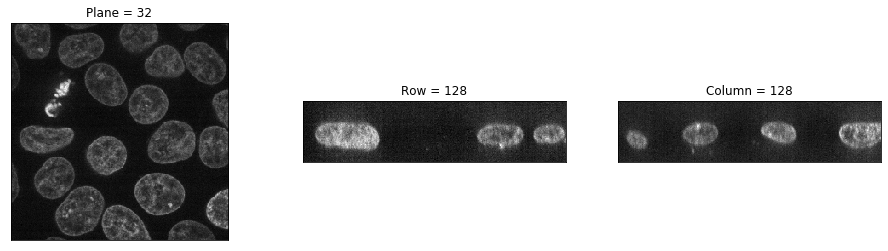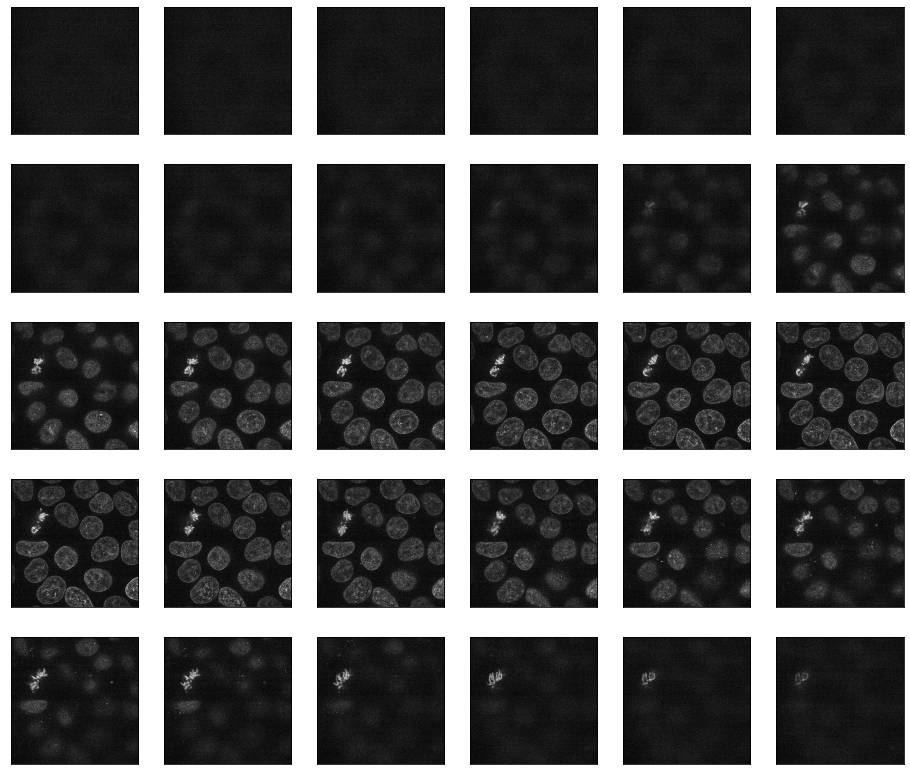In :
%matplotlib inline
from matplotlib import cm
from matplotlib import pyplot as plt
from mpl_toolkits.mplot3d.art3d import Poly3DCollection, Line3DCollection

import numpy as np

from scipy import ndimage as ndi

from skimage import (exposure, feature, filters, io, measure,
morphology, restoration, segmentation, transform,
util)


# Introduction to three-dimensional image processing¶

Images are represented as numpy arrays. A single-channel, or grayscale, image is a 2D matrix of pixel intensities of shape (row, column). We can construct a 3D volume as a series of 2D planes, giving 3D images the shape (plane, row, column). Multichannel data adds a channel dimension in the final position containing color information.

These conventions are summarized in the table below:

Image type Coordinates
2D grayscale (row, column)
2D multichannel (row, column, channel)
3D grayscale (plane, row, column)
3D multichannel (plane, row, column, channel)

Some 3D images are constructed with equal resolution in each dimension; e.g., a computer generated rendering of a sphere. Most experimental data captures one dimension at a lower resolution than the other two; e.g., photographing thin slices to approximate a 3D structure as a stack of 2D images. The distance between pixels in each dimension, called spacing, is encoded in a tuple and is accepted as a parameter by some skimage functions and can be used to adjust contributions to filters.

## Input/Output and display¶

Three dimensional data can be loaded with skimage.io.imread. The data for this tutorial was provided by the Allen Institute for Cell Science. It has been downsampled by a factor of 4 in the row and column dimensions to reduce computational time.

In :
data = io.imread("../images/cells.tif")

print("shape: {}".format(data.shape))
print("dtype: {}".format(data.dtype))
print("range: ({}, {})".format(data.min(), data.max()))

shape: (60, 256, 256)
dtype: float64
range: (0.0, 1.0)


The distance between pixels was reported by the microscope used to image the cells. This spacing information will be used to adjust contributions to filters and helps decide when to apply operations planewise. We've chosen to normalize it to 1.0 in the row and column dimensions.

In :
# The microscope reports the following spacing
original_spacing = np.array([0.2900000, 0.0650000, 0.0650000])

# We downsampled each slice 4x to make the data smaller
rescaled_spacing = original_spacing * [1, 4, 4]

# Normalize the spacing so that pixels are a distance of 1 apart
spacing = rescaled_spacing / rescaled_spacing

print("microscope spacing: {}\n".format(original_spacing))
print("after rescaling images: {}\n".format(rescaled_spacing))
print("normalized spacing: {}\n".format(spacing))

microscope spacing: [0.29  0.065 0.065]

after rescaling images: [0.29 0.26 0.26]

normalized spacing: [1.11538462 1.         1.        ]



To illustrate (no need to read the following cell; execute to generate illustration).

In :
# To make sure we all see the same thing
np.random.seed(0)

image = np.random.random((8, 8))
image_rescaled = transform.downscale_local_mean(image, (4, 4))

f, (ax0, ax1) = plt.subplots(1, 2)

ax0.imshow(image, cmap='gray')
ax0.set_xticks([])
ax0.set_yticks([])
centers = np.indices(image.shape).reshape(2, -1).T
ax0.plot(centers[:, 0], centers[:, 1], '.r')

ax1.imshow(image_rescaled, cmap='gray')
ax1.set_xticks([])
ax1.set_yticks([])
centers = np.indices(image_rescaled.shape).reshape(2, -1).T
ax1.plot(centers[:, 0], centers[:, 1], '.r');Back to our original data, let's try visualizing the image with skimage.io.imshow.

In :
try:
io.imshow(data, cmap="gray")
except TypeError as e:
print(str(e))

Invalid shape (60, 256, 256) for image dataskimage.io.imshow can only display grayscale and RGB(A) 2D images. We can use skimage.io.imshow to visualize 2D planes. By fixing one axis, we can observe three different views of the image.

In :
def show_plane(ax, plane, cmap="gray", title=None):
ax.imshow(plane, cmap=cmap)
ax.set_xticks([])
ax.set_yticks([])

if title:
ax.set_title(title)

In :
_, (a, b, c) = plt.subplots(nrows=1, ncols=3, figsize=(16, 4))

show_plane(a, data, title="Plane = 32")
show_plane(b, data[:, 128, :], title="Row = 128")
show_plane(c, data[:, :, 128], title="Column = 128")Three-dimensional images can be viewed as a series of two-dimensional functions. The display helper function displays 30 planes of the provided image. By default, every other plane is displayed.

In :
def slice_in_3D(ax, i):
# From:
# https://stackoverflow.com/questions/44881885/python-draw-3d-cube

import numpy as np
from mpl_toolkits.mplot3d.art3d import Poly3DCollection, Line3DCollection

Z = np.array([[0, 0, 0],
[1, 0, 0],
[1, 1, 0],
[0, 1, 0],
[0, 0, 1],
[1, 0, 1],
[1, 1, 1],
[0, 1, 1]])

Z = Z * data.shape

r = [-1,1]

X, Y = np.meshgrid(r, r)
# plot vertices
ax.scatter3D(Z[:, 0], Z[:, 1], Z[:, 2])

# list of sides' polygons of figure
verts = [[Z, Z, Z, Z],
[Z, Z, Z, Z],
[Z, Z, Z, Z],
[Z, Z, Z, Z],
[Z, Z, Z, Z],
[Z, Z, Z, Z],
[Z, Z, Z, Z]]

# plot sides
Poly3DCollection(verts, facecolors=(0, 1, 1, 0.25), linewidths=1,
edgecolors='darkblue')
)

verts = np.array([[[0, 0, 0],
[0, 0, 1],
[0, 1, 1],
[0, 1, 0]]])
verts = verts * (60, 256, 256)
verts += [i, 0, 0]

facecolors='magenta', linewidths=1, edgecolors='black'))

ax.set_xlabel('plane')
ax.set_ylabel('col')
ax.set_zlabel('row')

# Auto-scale plot axes
scaling = np.array([getattr(ax, 'get_{}lim'.format(dim))() for dim in 'xyz'])
ax.auto_scale_xyz(*[[np.min(scaling), np.max(scaling)]] * 3)

#plt.show()

In :
from ipywidgets import interact

def slice_explorer(data, cmap='gray'):
N = len(data)

@interact(plane=(0, N - 1))
def display_slice(plane=34):
fig, ax = plt.subplots(figsize=(20, 5))

show_plane(ax, data[plane], title="Plane {}".format(plane), cmap=cmap)
slice_in_3D(ax_3D, plane)

plt.show()

return display_slice

In :
slice_explorer(data);

In :
def display(im3d, cmap="gray", step=2):
_, axes = plt.subplots(nrows=5, ncols=6, figsize=(16, 14))

vmin = im3d.min()
vmax = im3d.max()

for ax, image in zip(axes.flatten(), im3d[::step]):
ax.imshow(image, cmap=cmap, vmin=vmin, vmax=vmax)
ax.set_xticks([])
ax.set_yticks([])

In :
display(data)## Exposure¶

skimage.exposure contains a number of functions for adjusting image contrast. These functions operate on pixel values. Generally, image dimensionality or pixel spacing does not need to be considered.

Gamma correction, also known as Power Law Transform, brightens or darkens an image. The function $O = I^\gamma$ is applied to each pixel in the image. A gamma < 1 will brighten an image, while a gamma > 1 will darken an image.

In :
# Helper function for plotting histograms.
def plot_hist(ax, data, title=None):
ax.hist(data.ravel(), bins=256)
ax.ticklabel_format(axis="y", style="scientific", scilimits=(0, 0))

if title:
ax.set_title(title)

In :
gamma_low_val = 0.5

gamma_high_val = 1.5